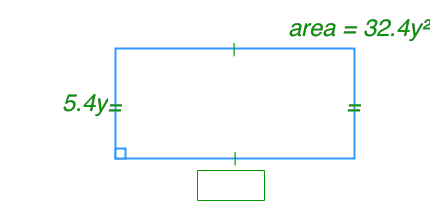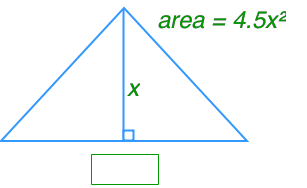# Multiplying and dividing monomials#### All in One Place

Everything you need for better grades in university, high school and elementary.#### Learn with Ease

Made in Canada with help for all provincial curriculums, so you can study in confidence.#### Instant and Unlimited Help

0/2
##### Intros
###### Lessons
1. How to multiply monomials?
2. How to divide monomials?
0/18
##### Examples
###### Lessons
1. Model and calculate each multiplication.
1. $\left( {6x} \right)\left( {2x} \right)$
2. $\left( { - 4y} \right)\left( {3y} \right)$
3. $\left( x \right)\left( { - 5x} \right)$
4. $\left( { - 2x} \right)\left( {7x} \right)$
2. Multiply.
1. $\left( x \right)\left( { - 4x} \right)$
2. $\left( {5x} \right)\left( {3x} \right)$
3. $\left( { - 2x} \right)\left( { - 6x} \right)$
4. $\left( { - 3.1x} \right)\left( { - 4x} \right)$
5. $\left( { - \frac{3}{4}x} \right)\left( {16x} \right)$
6. $\left( { - 8x} \right)\left( {2.3y} \right)$
3. Divide.
1. $\frac{{144x}}{6}$
2. $\frac{{84xy}}{{4xy}}$
3. $\frac{{9{x^2}}}{x}$
4. $\frac{{18xy}}{{3x}}$
5. $\frac{9.6{p^2}}{ - 8p}$
6. $\frac{{ - 25x}}{{ - 5x}}$
4. Find the missing dimension.

1.2.0%
##### Practice
###### Topic Notes
In this section, we will practice multiplying and dividing polynomials. Furthermore, we will use polynomial multiplication and division to solve unknowns such as, side length, and surface area, of polygons.